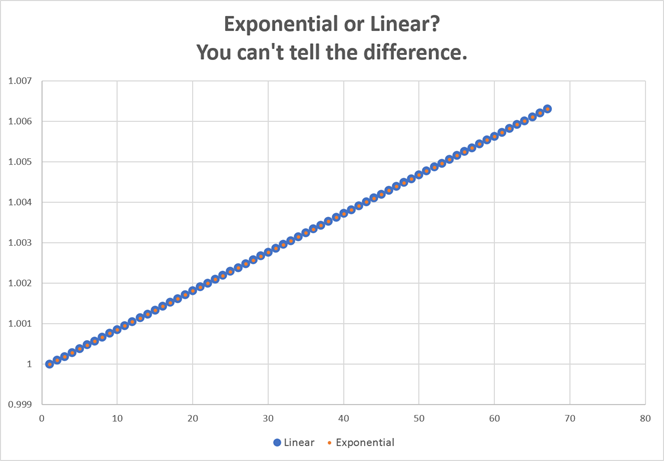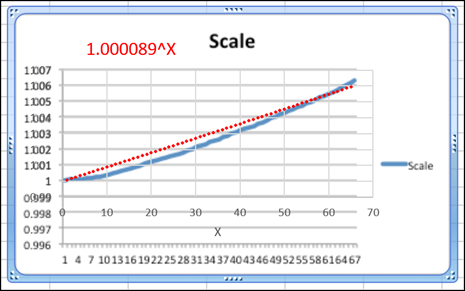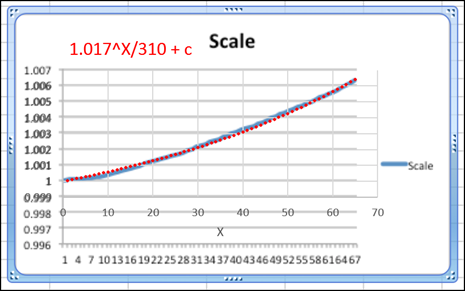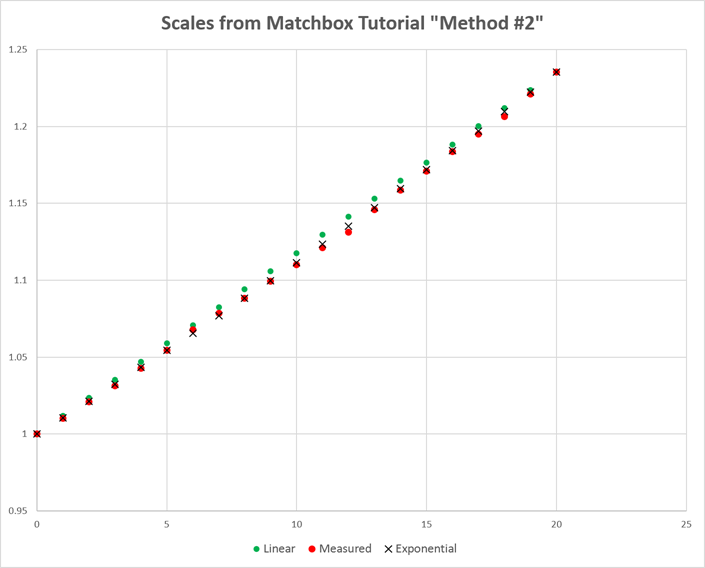## Telecentric Scanner-Nikkor ED LENS: Nikon 8000 ED lens

Have questions about the equipment used for macro- or micro- photography? Post those questions in this forum.

Moderators: ChrisR, Chris S., Pau, rjlittlefield

Lou Jost
Posts: 4423
Joined: Fri Sep 04, 2015 7:03 am
Contact:
Mike, the scale factor is cumulative, as Rik has told us somewhere on the forum. So it can be misleading to use the end number in comparisons; this will depend not only on the telecentricity but also on the depth of the stack.

Can you plot y(x+1) divided by y(x) for each point? That should be a more nearly level line, which won't depend so strongly on the stack depth.

In words, for each value of x between 1 and 66, take the scale factor for x and the scale factor for the step after x, and divide them, and plot that number instead of the raw scale factor for that value of x.

RDolz
Posts: 89
Joined: Mon Aug 28, 2017 9:32 am
Location: Valencia (Spain)
This of the telecentricity is a concept that resists me ...
Lou, Mike, after studying your contributions, I have focused the measure of telecentricity in another way. I have normalized the variation of scale with respect to the displacement of the Z axis in each image.

Telecentricity is measured in degrees. So, for example, a lens with an object-space telecentricity of 0.2 degrees will accept light at angles of up to 0.2 degrees away from parallel to the optical axis.

A lens whose telecentricity is 0.1 degrees is considered very good. This means that the error for a displacement of 1 mm would be 1.7 micron.

What I have done is measure this angle from the Zerene scale data, assuming that the scale variation data is exactly assimilable to pixel variation.

The first step is to transform the scale value into pixels. Next I turn the variation into pixels into variation in mm of the horizontal field of view.
I have considered only half the value in pixels, since the calculation of the variation of scale is for the whole image, and the error in the telecentricity is symmetric to the optical axis (is it correct?)

Finally I compare the variation of the horizontal field of view with the axis travel. With these values I calculate the telecentricity angle.

With these premises, I have obtained, for the Coolscan 8000, a telecentricity that ranges between 0.06º and 0.25º, depending on the stacking. This means that the error for a displacement of 1 mm would oscillate between 1 and 4.3 micron.

I have to emphasize that the object, its planarity, its morphology ... etc, make this value change.

This is a graph that I have obtained from a single stacking.The best
Last edited by RDolz on Wed Dec 26, 2018 3:26 am, edited 1 time in total.
Ramón Dolz

rjlittlefield
Posts: 20857
Joined: Tue Aug 01, 2006 8:34 am
Location: Richland, Washington State, USA
Contact:
I've been struggling to figure out how to explain this clearly and concisely.

Let's try this for a quick summary:

1. The important number for focus stacking is scale change per DOF. A larger angle in degrees can be tolerated at higher magnification and with wider apertures, both of which give less DOF in proportion to frame width.

2. How you compute scale change makes no significant difference. If one image is scale A=1.0002 and the next one is B=1.0003, then to very high accuracy the change in scale is 0.0001 = 0.01% = 1 part in 10,000 no matter whether you compute it as B-A or (B/A)-1. Similarly if A and B are the endpoint scales of N frames, then it makes no significant difference whether you calculate scale change per frame as (B-A)/(N-1) or (B/A)^(1/(N-1)).

3. The obvious nonlinearity shown in Mike's graph is not an exponential. That is, it's not due to repeated multiplication. Instead, it is an artifact that results from Zerene Stacker's alignment algorithm getting misled by changes in image appearance that are not really changes in scale, but to the computation look like they are.

--------------------- end summary ---------------------

With that summary in place, now let me fill in some more detail.

The numbers reported by Zerene Stacker are the entire transformation that gets applied to each image before merging it into the rendered composite. The numbers are typically computed by comparing adjacent frames, but the numbers themselves are cumulative.

When optical scaling is the only factor in play, the formally correct model is exponential, K^X with frame number X and fixed K which depends on the optics and the step size.

HOWEVER...

For lenses that are nearly telecentric, the total amount of scale change due to geometric optics is so small that there is vanishingly little difference between exponential and linear models.

Here is a graph of K^X and 1+M*X, for K and M computed so that the endpoints match Mike's data. Note that the red exponential points lie very neatly in the centers of the blue linear points. Numerically, the maximum difference between these two models is less than 5 parts per million. To repeat myself, "there is vanishingly little difference between exponential and linear models" in this range.In contrast, Mike showed:
Here's an example of what I'm referring to. Using:

Y = K^X, I get ~ 1.000089 for K

<graph overlayed below>
Unfortunately Mike's data leads easily into an all too common trap: assume a functional form, compute a fit, then do not look to see how well the fit actually works.

If we do look, what we see is that the fit is not exactly stellar. (And as we saw above, a linear fit would have looked almost exactly the same.)The difficulty is that the curve shown by Mike's data is not really an exponential even though visually it looks like it is. Yes, that upward concavity looks very much like graphs that we've all seen in books, but when we pay attention to the values on the Y axis and compute what the numbers in between would have to be if it were exponential, they don't work. Over this range of values, an exponential function is almost indistinguishable from linear.

We could certainly create an exponential-ish function that fits Mike's data, but that "ish" is important. Here's one that fits pretty well:But the problem here is that those three numbers -- 1.017, 310, and c -- have no physical meaning. They don't relate to anything in the optics. They're just what's needed to match the endpoints and the curvature of Mike's data. In fact I'm pretty confident that if Mike re-ran his test with a significantly different type of subject and scene, then he'd get a curve with different numbers and probably even a different shape.

To accurately measure telecentricity requires a careful test with a special setup: a planar subject that is imaged equal distances in front and behind perfect focus, so that nothing appears different between the two images except their size.

Any other sort of measurement will be less accurate. As Ramon has noted, "I have to emphasize that the object, its planarity, its morphology ... etc, make this value change."
RDolz wrote:I have normalized the variation of scale with respect to the displacement of the Z axis in each image.
This is on the right track in some respects, but not all.

One good aspect is that it removes the effect of any arbitrary change of step size. Suppose for example that some particular lens has 0.01% change per 10 microns. Then that same lens will have 0.02% change per 20 microns but only 0.005% change per 5 microns. If I can arbitrarily choose to measure with 10 or 20 or 5 microns step size, then which number should I report? Normalizing scale change by displacement eliminates that ambiguity.

But on the flip side, one bad aspect is that it hides the importance of DOF. Under reasonable assumptions, the ratio of DOF to frame width drops roughly in proportion to the magnification. As a result, you can tolerate a lot larger angles when working at 10X versus 1X. So, a lens that has 0.2 degrees telecentricity is either OK or not, depending on what magnification we're talking about.

--Rik

Edit: correct some math in the last paragraph
Last edited by rjlittlefield on Thu Dec 27, 2018 1:16 am, edited 1 time in total.

Lou Jost
Posts: 4423
Joined: Fri Sep 04, 2015 7:03 am
Contact:
Thanks for that, Rik.

You say in Point 2:
it makes no significant difference whether you calculate scale change per frame as (B-A)/(N-1) or (B/A)^(1/(N-1)).

but people might miss your qualifying comment:
For lenses that are nearly telecentric, the total amount of scale change due to geometric optics is so small that there is vanishingly little difference between exponential and linear models.
We should use a method that doesn't assume the lens is telecentric, so the exponential model should be the default, right?

You didn't comment on my suggestion:
I've been quantifying telecentricity by measuring the ratio of the scale factors between successive frames, using the step sizes in Rik's Zerene table. This is insensitive to stack depth. And if you do it in different parts of the stack, you can find out if the central region is more telecentric than the margins.
This gets at standardizing step size, and variations across the frame. Is this a reasonable approach?

mawyatt
Posts: 2473
Joined: Thu Aug 22, 2013 6:54 pm
Location: Clearwater
Rik, Lou,

The scale graph I presented was from a 6 tile S&S session with a subject (chip) titled back and angled so the image had a varying "depth" of up to ~2mm. Thus not an attempt to evaluate lens telecentricity, in fact the only purpose of this session was to evaluate the S&S software and hardware. The subject chip was about as far from normal to the optical axis as possible, this was to create a somewhat 3D effect, rather than the usual planar effect.

Here's a couple other scale graphs from other tiles. Note the difference in "appearance" between these, certainly indicating this was not a good stacking session to evaluate telecentricity!!

1st GraphOthersBest & Happy Holidays,
Research is like a treasure hunt, you don't know where to look or what you'll find!
~Mike

rjlittlefield
Posts: 20857
Joined: Tue Aug 01, 2006 8:34 am
Location: Richland, Washington State, USA
Contact:
Lou Jost wrote:We should use a method that doesn't assume the lens is telecentric, so the exponential model should be the default, right?
Let me show some more data before answering this question.

This data comes from the stack that I used for the Zerene Stacker "Tutorial #2: Using a Macro Lens on a DSLR", the second method "Focusing by Moving the Camera or Subject". You can review the setup HERE. Very briefly, it consists of an ordinary macro lens, set on 1:1 with APS-C sensor, stepped at 0.5 mm using an inexpensive manual rail. It's very not telecentric: over 23% scale change in 21 frames.

I have exported the scale factors from the project file, pulled them into Excel, and run linear and exponential curves through the endpoints.Numerically, the "average" change of scale (B-A)/N is 1.18% per step, while the exponential change of scale (B/A)^(1/N) is 1.01% per step.

Note that both of these numbers are roughly 50 times larger than any lens that we would consider suitable for use as telecentric. So we're way outside the ballpark of being telecentric, and still the linear and exponential fits are giving pretty close to the same number.

Further, the relative error between the linear and exponential fits decreases in direct proportion to the scale change. So by the time we get in the vicinity of telecentric, instead of 1.01% versus 1.18%, we're looking at 0.0200% versus 0.0201% for the exponential versus linear fits. Or in the case of Ramón's data, 0.9946 over 13 frames, it's a matter of 0.000411 per step for exponential versus 0.000413 for linear. Such small differences due to computational method are surely far less than errors contributed by several other causes.

So now, to answer the question...

If you're comfortable using an exponential model, then you should do that. You will sleep better, not having to worry whether you're "doing the right thing".

However, you should also be comfortable with anybody else who computes their number using a linear model. If the number is small enough to indicate that the lens is a candidate, then it will also be close enough that linear versus exponential makes no real difference.
Lou Jost wrote:
I've been quantifying telecentricity by measuring the ratio of the scale factors between successive frames, using the step sizes in Rik's Zerene table.
This gets at standardizing step size, and variations across the frame. Is this a reasonable approach?
This part sounds like what I've phrased as "scale change per DOF", using a 1/4-lambda criterion for DOF. Yes, very reasonable.
And if you do it in different parts of the stack, you can find out if the central region is more telecentric than the margins.
This part makes me nervous. I agree it could work very well with a carefully chosen test target, say looking down the axis of a centered cone so that each in-focus slab would be a ring of detail in a sea of blur. With a more arbitrary subject, I would not trust the numbers because I would expect measured scale changes to be more driven by random OOF stuff than by lens geometry.
mawyatt wrote:Thus not an attempt to evaluate lens telecentricity, ... Here's a couple other scale graphs from other tiles. Note the difference in "appearance" between these, certainly indicating this was not a good stacking session to evaluate telecentricity!!
Noted, and thanks for the added examples.

I tried to phrase my concern carefully, "Mike's data leads easily into an all too common trap".

Perhaps I am overly sensitive to the issue, but I got the distinct feeling that linear versus exponential was being called out as an important aspect, and in my experience it just isn't. Other issues are far more important.

Personally, if I'm going to worry about any of my caveats being overlooked, it'll be the one I've posted earlier in this thread:
To accurately measure telecentricity requires a careful test with a special setup: a planar subject that is imaged equal distances in front and behind perfect focus, so that nothing appears different between the two images except their size.

Measurements taken in any other scenario will be less accurate, perhaps seriously so depending on scene geometry and illumination.

--Rik

Lou Jost
Posts: 4423
Joined: Fri Sep 04, 2015 7:03 am
Contact:
OK, makes sense. But I think it may be worth emphasizing one of your points. The numbers are cumulative. So a really important part of this analysis is the need to compare two neighboring values (recognizing that the number will vary depending on which details are sharp at the chosen point in the stack; it might be worth choosing a place in the stack where central details are the ones that are sharp, and another place where edge details are the ones that are sharp), not the scale factor itself as given in Zerene. And it is important to standardize step size according to depth of field.

For the comparison of neighboring values, it is no harder to take their ratio than their difference, and if the lens is far from telecentric, taking the difference could lead to a significant error. So we might as well do it right. But I see that this is not the most important point.

mawyatt
Posts: 2473
Joined: Thu Aug 22, 2013 6:54 pm
Location: Clearwater
Lou, Rik,

I just did a little algebra that follows:

Successive Scale samples are S1 and S2, where S1 = 1+ s1 and S2 = 1+ s2.

The Difference Scale is S1-S2 or s1-s2

The Ratio Scale is S1/S2 or (1+s1)/(1+s2). If I multiply both Den and Num by 1-s2 then,

(1+s1)*(1-s2)/(1+s2)*(1-s2) or (1+s1-s2-s1s2)/(1-s2*s2)

If s1 and s2 are small then s1s2~0 and s2*s2~0

So Ratio is 1+s1-s2, same a 1 + Difference (for small s1 and s2).

Best,
Research is like a treasure hunt, you don't know where to look or what you'll find!
~Mike

etalon
Posts: 67
Joined: Tue Jan 05, 2016 5:26 am
Location: Germany
Contact:
Hello Ramon,

thanks for all those tests and informations. I have one more question concerning your setup: As I saw on your picture, you use the scanner nikkor 8000 in reversed mode (like Robert in his setup, too). Is there any atvantage in reversed mode vs normal orientation? Have you ever tried the lens in normal orientation?

Cheers,
Markus

RDolz
Posts: 89
Joined: Mon Aug 28, 2017 9:32 am
Location: Valencia (Spain)
Hi Markus,

Yes, I mounted it in that direction because when mounting the diaphragm to achieve the telecentricity I noticed that the quality of the image was a bit better ....
Unfortunately I can not show you pictures, ... if possible, this week I make some captures and upload them.
Ramón Dolz

ray_parkhurst
Posts: 2655
Joined: Sat Nov 20, 2010 10:40 am
Location: Santa Clara, CA, USA
Contact:
Was the 9mm aperture required to achieve reasonable telecentricity? What would happen if you went with a 16mm aperture, giving ~f7 effective?

rjlittlefield
Posts: 20857
Joined: Tue Aug 01, 2006 8:34 am
Location: Richland, Washington State, USA
Contact:
ray_parkhurst wrote:Was the 9mm aperture required to achieve reasonable telecentricity? What would happen if you went with a 16mm aperture, giving ~f7 effective?
One limitation is that the size of the telecentric field shrinks as the aperture gets wider. The telecentric field cannot be any larger than the diameter of the lens minus the diameter of the entrance cone where it enters the lens. Using a thin lens model, the diameter of the entrance cone at the lens will be (m+1)/m times the diameter of the added aperture. At say m=1, if you subtract 2*16 mm from the diameter of the lens, how much is left?

--Rik

etalon
Posts: 67
Joined: Tue Jan 05, 2016 5:26 am
Location: Germany
Contact:
Hi Ramon,

thank you very much. Your pics would be much appreciated. Would there also be an atvantage in reverse mode, if I use that lens not in telecentric mode?

I have a Nikon coolscan 4000 lens, and I found, that the pics would be better in normal orientation. But that is only my opinion and I can‘t proof it...

Cheers,
Markus

Lou Jost
Posts: 4423
Joined: Fri Sep 04, 2015 7:03 am
Contact:
Regarding the mounting direction, we should always specify the magnification.

This lens is not perfectly symmetric so the direction should matter, depending on the desired magnification. When I was getting this lens out of the scanner, I wrote this:
"I'm dissecting my Coolscan 8000 now. The line sensor is approx 60 mm long and the distance from the rear flange of the lens to this sensor is about 130 mm. [Edit-- I bought a decent ruler and I find that the flange-to-sensor distance is 128mm.] The distance from the front flange to the subject is very roughly 152 mm. So this lens has a huge image circle and is working at close to 1:1 since medium format film is 60mm wide."

I think ray has since pointed out that the PN105 also has such a large image circle. But the Coolscan 4000 and 5000 do not.

http://www.photomacrography.net/forum/v ... 6&start=15

http://www.photomacrography.net/forum/v ... n&start=15

I was impressed at the time, and got quite excited about scanners from then on.

etalon
Posts: 67
Joined: Tue Jan 05, 2016 5:26 am
Location: Germany
Contact:
Hi Lou,

thanks for your experience. I want to take the lens for 1:1. How would you use the lens for that, normal or reversed?
Do you know, how far the magnification can be varied from 1:1, before the resolution degrade visible with an mft sensor?
Cheers,
Markus

P.S. I use the coolscan 4000 lens at 1,3x.